# RD Sharma Solutions For Class 7 Maths Exercise 3.2 Chapter 3 Decimals

The exercise-wise solutions are depicted in an interactive manner to help students with their exam preparation. RD Sharma Solutions for Class 6 Maths Chapter 3 Decimals Exercise 3.2 are provided here. Students can download this PDF from the provided links. The expert faculty at BYJU’S provides students with PDF of solutions to solve the problems according to the CBSE syllabus. The solutions for exercise wise problems are prepared after vast research is conducted on each topic. The step-wise solutions prepared by subject experts help students to solve problems comfortably.

By practising the RD Sharma Solutions for Class 7, students will be able to grasp the concepts correctly. Conceptual knowledge is crucial as some of the topics are continued in higher classes.

## Download the PDF of RD Sharma Solutions For Class 7 Maths Chapter 3 – Decimals Exercise 3.2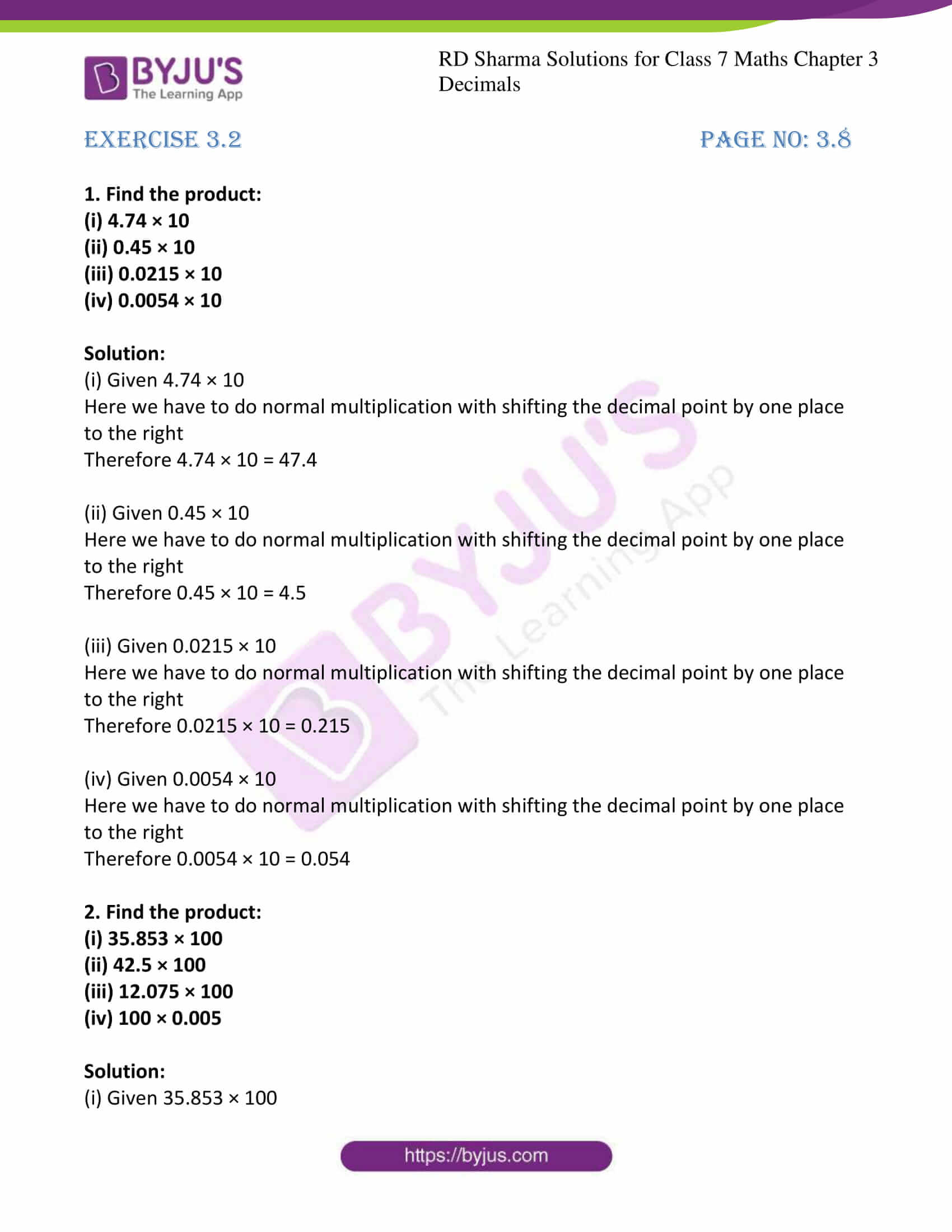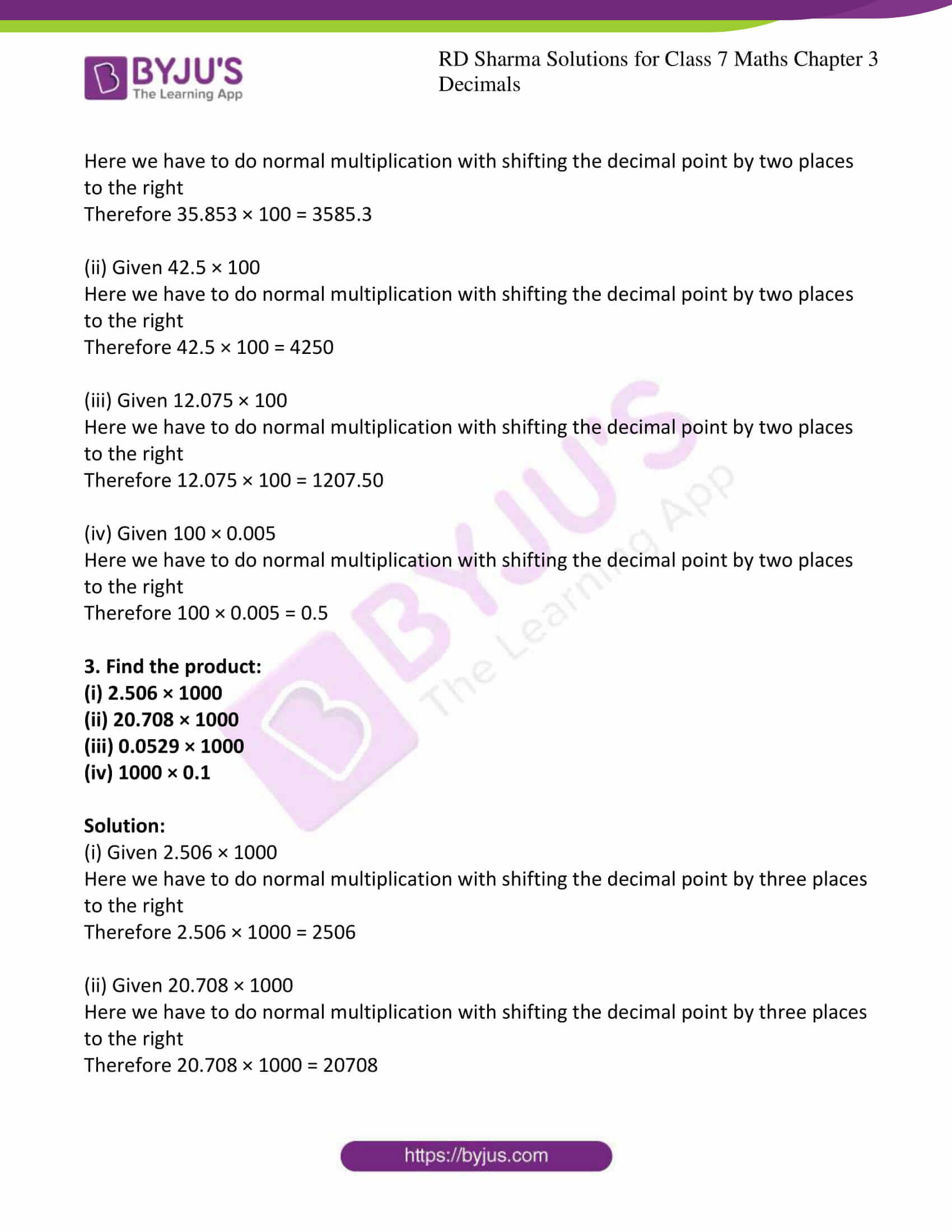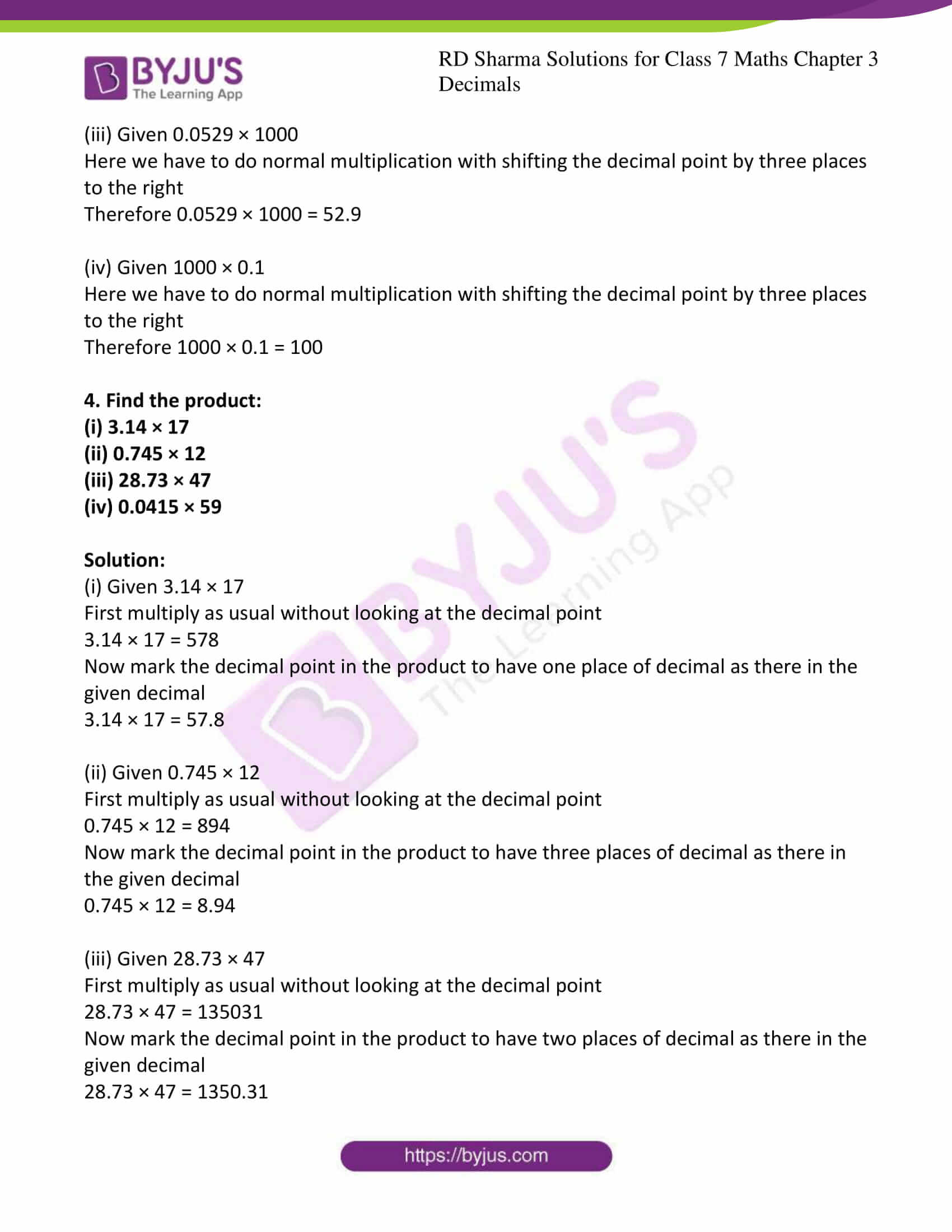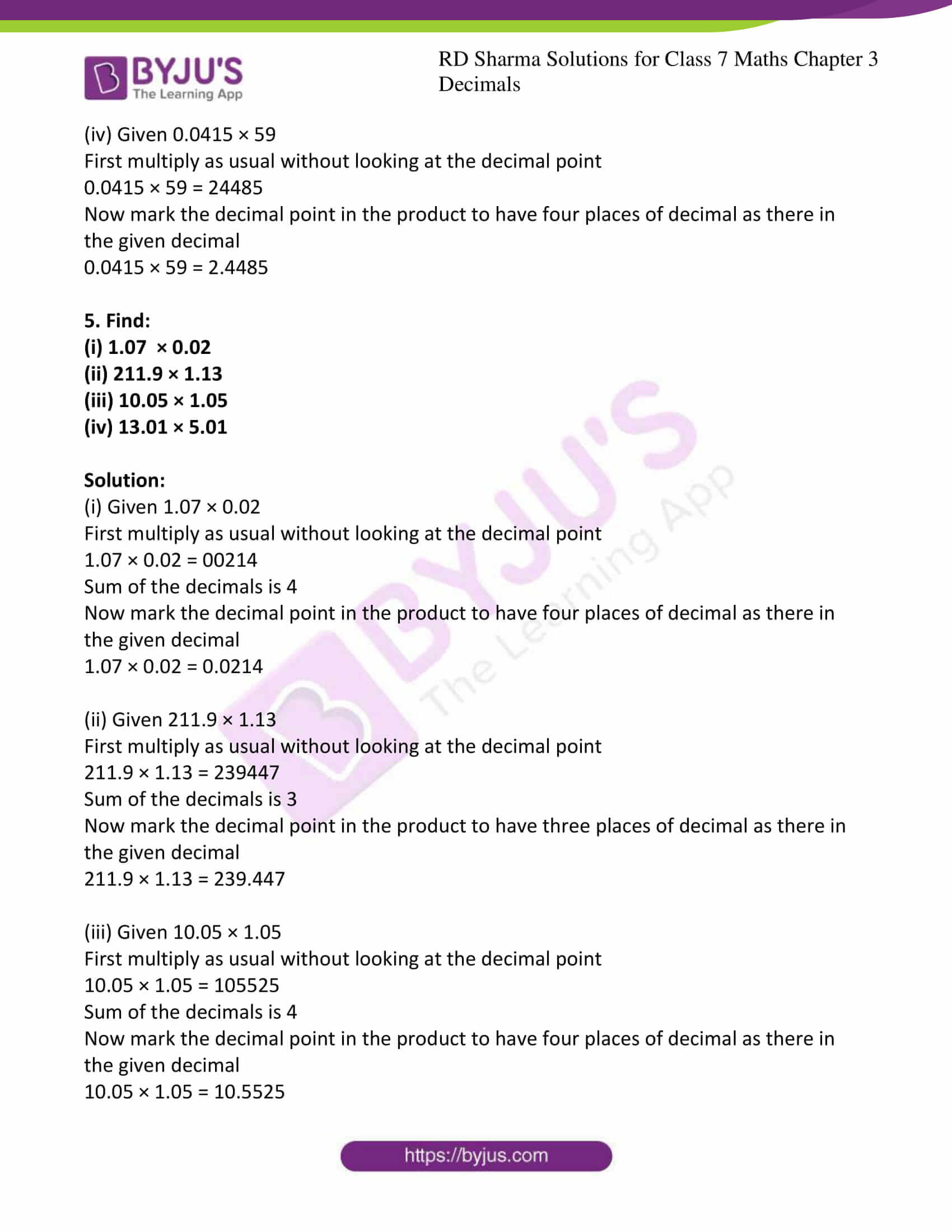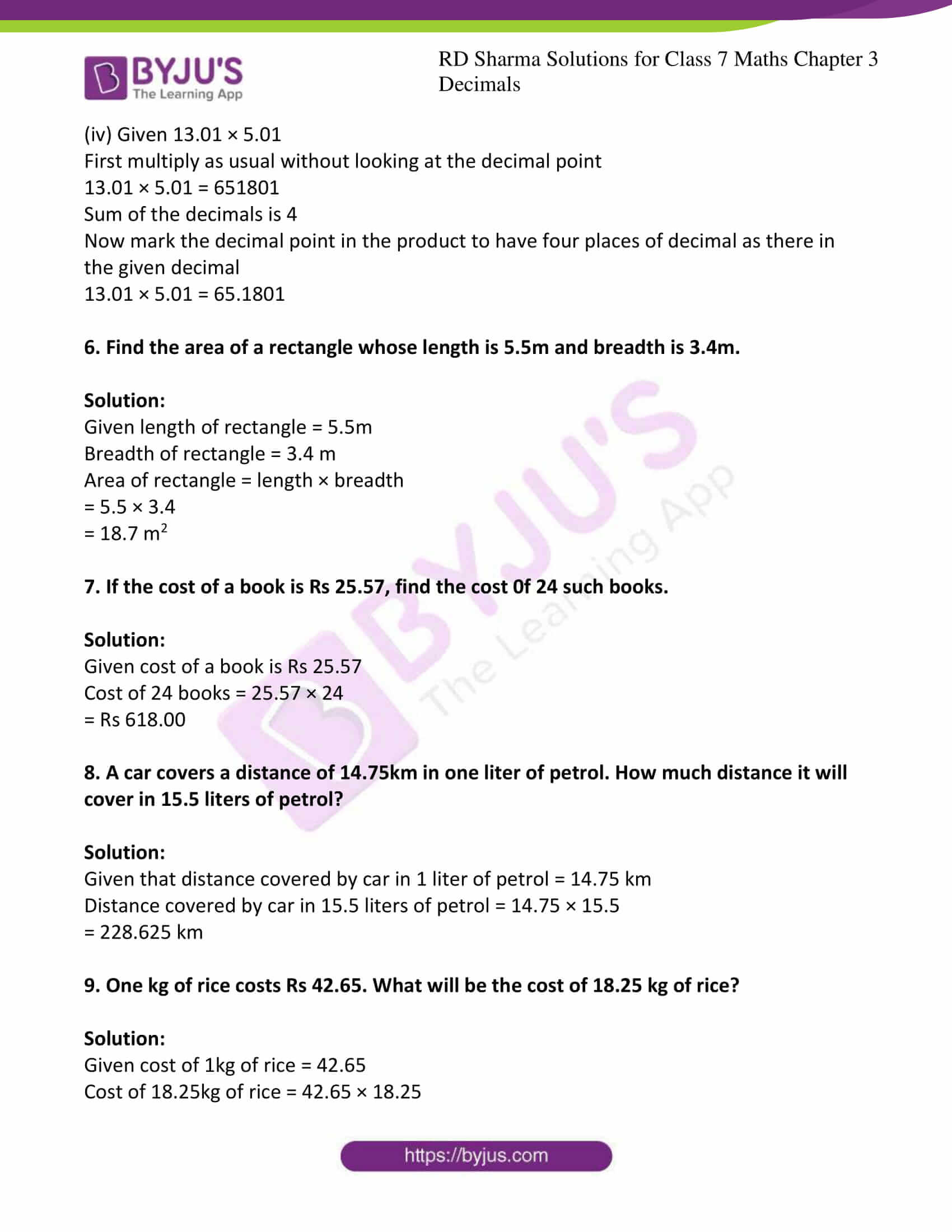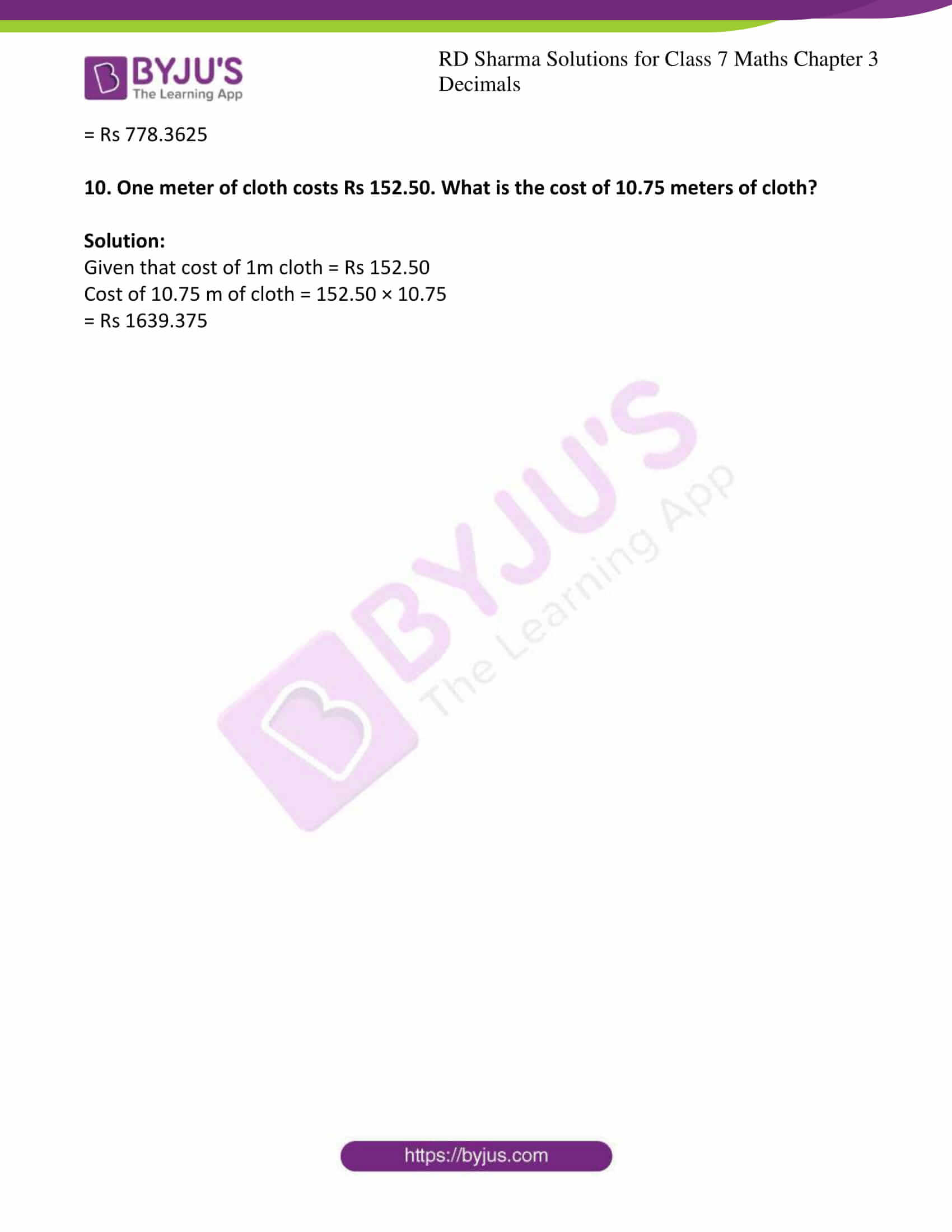### Access answers to Maths RD Sharma Solutions For Class 7 Chapter 3 – Decimals Exercise 3.2

1. Find the product:

(i) 4.74 × 10

(ii) 0.45 × 10

(iii) 0.0215 × 10

(iv) 0.0054 × 10

Solution:

(i) Given 4.74 × 10

Here we have to do normal multiplication with shifting the decimal point by one place to the right

Therefore 4.74 × 10 = 47.4

(ii) Given 0.45 × 10

Here we have to do normal multiplication with shifting the decimal point by one place to the right

Therefore 0.45 × 10 = 4.5

(iii) Given 0.0215 × 10

Here we have to do normal multiplication with shifting the decimal point by one place to the right

Therefore 0.0215 × 10 = 0.215

(iv) Given 0.0054 × 10

Here we have to do normal multiplication with shifting the decimal point by one place to the right

Therefore 0.0054 × 10 = 0.054

2. Find the product:

(i) 35.853 × 100

(ii) 42.5 × 100

(iii) 12.075 × 100

(iv) 100 × 0.005

Solution:

(i) Given 35.853 × 100

Here we have to do normal multiplication with shifting the decimal point by two places to the right

Therefore 35.853 × 100 = 3585.3

(ii) Given 42.5 × 100

Here we have to do normal multiplication with shifting the decimal point by two places to the right

Therefore 42.5 × 100 = 4250

(iii) Given 12.075 × 100

Here we have to do normal multiplication with shifting the decimal point by two places to the right

Therefore 12.075 × 100 = 1207.50

(iv) Given 100 × 0.005

Here we have to do normal multiplication with shifting the decimal point by two places to the right

Therefore 100 × 0.005 = 0.5

3. Find the product:

(i) 2.506 × 1000

(ii) 20.708 × 1000

(iii) 0.0529 × 1000

(iv) 1000 × 0.1

Solution:

(i) Given 2.506 × 1000

Here we have to do normal multiplication with shifting the decimal point by three places to the right

Therefore 2.506 × 1000 = 2506

(ii) Given 20.708 × 1000

Here we have to do normal multiplication with shifting the decimal point by three places to the right

Therefore 20.708 × 1000 = 20708

(iii) Given 0.0529 × 1000

Here we have to do normal multiplication with shifting the decimal point by three places to the right

Therefore 0.0529 × 1000 = 52.9

(iv) Given 1000 × 0.1

Here we have to do normal multiplication with shifting the decimal point by three places to the right

Therefore 1000 × 0.1 = 100

4. Find the product:

(i) 3.14 × 17

(ii) 0.745 × 12

(iii) 28.73 × 47

(iv) 0.0415 × 59

Solution:

(i) Given 3.14 × 17

First multiply as usual without looking at the decimal point

3.14 × 17 = 578

Now mark the decimal point in the product to have one place of decimal as there in the given decimal

3.14 × 17 = 57.8

(ii) Given 0.745 × 12

First multiply as usual without looking at the decimal point

0.745 × 12 = 894

Now mark the decimal point in the product to have three places of decimal as there in the given decimal

0.745 × 12 = 8.94

(iii) Given 28.73 × 47

First multiply as usual without looking at the decimal point

28.73 × 47 = 135031

Now mark the decimal point in the product to have two places of decimal as there in the given decimal

28.73 × 47 = 1350.31

(iv) Given 0.0415 × 59

First multiply as usual without looking at the decimal point

0.0415 × 59 = 24485

Now mark the decimal point in the product to have four places of decimal as there in the given decimal

0.0415 × 59 = 2.4485

5. Find:

(i) 1.07 × 0.02

(ii) 211.9 × 1.13

(iii) 10.05 × 1.05

(iv) 13.01 × 5.01

Solution:

(i) Given 1.07 × 0.02

First multiply as usual without looking at the decimal point

1.07 × 0.02 = 00214

Sum of the decimals is 4

Now mark the decimal point in the product to have four places of decimal as there in the given decimal

1.07 × 0.02 = 0.0214

(ii) Given 211.9 × 1.13

First multiply as usual without looking at the decimal point

211.9 × 1.13 = 239447

Sum of the decimals is 3

Now mark the decimal point in the product to have three places of decimal as there in the given decimal

211.9 × 1.13 = 239.447

(iii) Given 10.05 × 1.05

First multiply as usual without looking at the decimal point

10.05 × 1.05 = 105525

Sum of the decimals is 4

Now mark the decimal point in the product to have four places of decimal as there in the given decimal

10.05 × 1.05 = 10.5525

(iv) Given 13.01 × 5.01

First multiply as usual without looking at the decimal point

13.01 × 5.01 = 651801

Sum of the decimals is 4

Now mark the decimal point in the product to have four places of decimal as there in the given decimal

13.01 × 5.01 = 65.1801

6. Find the area of a rectangle whose length is 5.5m and breadth is 3.4m.

Solution:

Given length of rectangle = 5.5m

Breadth of rectangle = 3.4 m

Area of rectangle = length × breadth

= 5.5 × 3.4

= 18.7 m2

7. If the cost of a book is Rs 25.57, find the cost 0f 24 such books.

Solution:

Given cost of a book is Rs 25.57

Cost of 24 books = 25.57 × 24

= Rs 618.00

8. A car covers a distance of 14.75km in one liter of petrol. How much distance it will cover in 15.5 liters of petrol?

Solution:

Given that distance covered by car in 1 liter of petrol = 14.75 km

Distance covered by car in 15.5 liters of petrol = 14.75 × 15.5

= 228.625 km

9. One kg of rice costs Rs 42.65. What will be the cost of 18.25 kg of rice?

Solution:

Given cost of 1kg of rice = 42.65

Cost of 18.25kg of rice = 42.65 × 18.25

= Rs 778.3625

10. One meter of cloth costs Rs 152.50. What is the cost of 10.75 meters of cloth?

Solution:

Given that cost of 1m cloth = Rs 152.50

Cost of 10.75 m of cloth = 152.50 × 10.75

= Rs 1639.375

### RD Sharma Solutions for Class 7 Maths Chapter – 3 Decimals Exercise 3.2

RD Sharma Solutions for Class 7 Maths Chapter 3 Decimals Exercise 3.2 has problems which are based on the multiplication of decimals. Some of the topics focused prior to this exercise are listed below.

• Multiplication of a decimal by 10, 100 and 1000
• Multiplication of a decimal by a whole number
• Multiplication of decimal by another decimal

Students are suggested to try solving the questions from RD Sharma book of Class 7 and then refer to these solutions to know the best way of approaching the different questions.